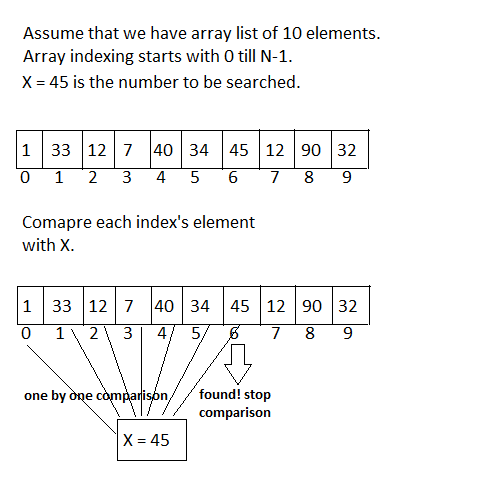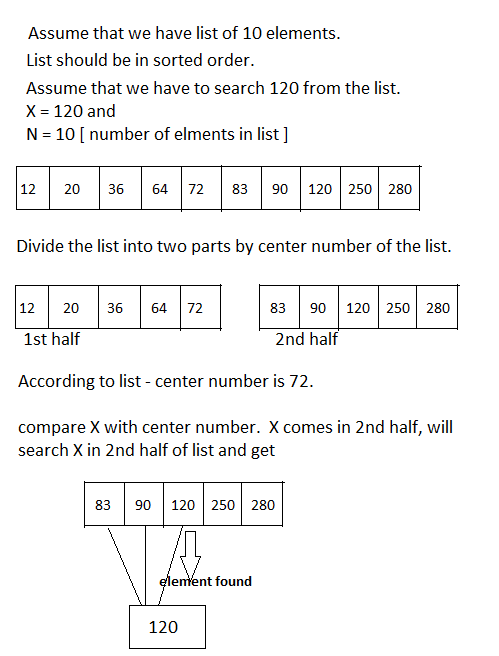We have gone through with Data Structure concepts and its operations implementations ex: insertion, deletion, traversing. Now will discuss about searching algorithms.

Searching is the technique to search any data element from list. Every search responses the status of searching successful or unsuccessful.

Here I will discuss mainly 2 types of searching techniques Linear or Sequential search and Binary search their efficiency and complexity level.

Linear Search or Sequential Search

Linear or sequential search is very basic form of searching elements.

Here we have a list of data element and will start searching element from start till end of the list until we search element.

It compares every list’s data element with to be searched value so that this search has O(n) complexity i.e. it takes n comparisons to search element.

This search technique works with both sorted and unsorted data values. If list is big then this kind of search is not feasible.
``````
Assume that we have N number of elements in list and we have to
search X element.

N = 10, X=45

Read the list from start
(if it’s an array then read from 0th index)

till N (Number of elements).

Compare every element of list with to be searched element X.

If X is found then give response in successful
otherwise unsuccessful.``````Linear Or Sequential Searching. ByTechAchievers.com
Linear Search Example In Java
``````
class ````LinearSearchingExample ````{

```` public static void main(String []args){````

int arrayList[] = {6,10,33,56,74,34,39,90,23,87};

int N = 10;

int X = 39;

System.out.println("Searching process ...\n");

for( int i=0;i<N; i++) {

if (arrayList [i] == X ) {

status = "found";

break;
}

}

System.out.println (" Element has been "+status);

}

}
``````

Binary Search

Binary search is another type of searching.
This search works on sorted list only. It is more efficient and faster than sequential search. Like sequential searching this search also returns searching status.

Binary search divides the list in to halves from the center of the list.

As the list is in order so that 1st part of list contains all elements that are less than center number and another half list contains all elements that are greater than center number.

Now according to the value of X (to be searched) we can search it in first half or in second half of the list so that search complexity is O(log N) i.e. less number of comparisons are required.

You can see that we don't need to search element in whole list.

``````
Assume that we have N number of elements in list and we have to
search X element.

N = 10, X=45

Now find the center index element from list.

Check X is less or greater than center number.

If X is less than center number, search X into 1st half of list.

If X is greater than center number, search X into 2nd half of list.

If X is found then give response in successful otherwise unsuccessful.``````Binary Searching. ByTechAchievers.com

Binary Search Example In Java

``````
public class ````BinarySearchingExample ````{

`````` public static void main(String []args) {
``````

int arrayList[] = {12,20,36,64,72,83,90,120,250,280};

int N = 10;

int X = 120;

System.out.println("Binary searching process starts...\n");

/* get center element of the list int

center_element = arrayList[N/2]; */

if(center_element == X) {

System.out.println(" X is the center_element of list \n" );

status ="found";

}

else{

if (X < center_element ) {

System.out.println(" X < center_element, search in 1st

half of list \n" );

/* passing list , X, initial index and center_element

index */

status = searchAlgorithm (arrayList,X,0,N/2);

}

else {

System.out.println(" X > center_element, search in 2nd

half of list \n" );

/* passing list , X, center_element index and List

limit */

status = searchAlgorithm (arrayList,X,N/2,N);

}

}

System.out.println (" Element has been "+status);

````}````

/* Method to search element in list */

``````public static String searchAlgorithm(int arrayList[],int X,

int index1, int index2) {
``````

for( int i=index1;i<index2 ; i++) {

if (arrayList [i] == X ) {

return "found";

}

}

````}````